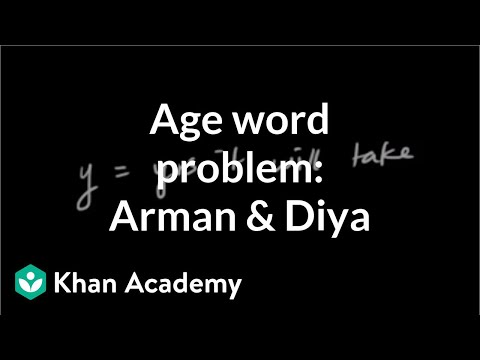Video

# X-intercept of a line (Full video)

Khan Academy

## Supporting grades: 8

Description: Sal determines the x-intercept of a linear equation from a graph. Afterwards, he checks his work by plugging values back into the equation. Created by Sal Khan. The graph of the line 2y plus 3x equals 7 is given right over here. The x-intercept is the x value when y is equal to 0, or it's the x value where our graph actually intersects the x-axis. So let's go turn to the equation to figure out the exact value.

You must log inorsign upif you want to:*

*Teacher Advisor is 100% free.

### Other videos you might be interested in### Solving Systems of Equations by Substitution (Full video)

#### Student Achievement Partners